# SAS Criterion in Triangles

Go back to  'Similar-Triangles'

We have an SAS axiom for congruence of two triangles. Do we have a similar criterion for similarity? Reflect for a moment on why there should be such a criterion.

SAS Criterion: If two sides of one triangle are respectively proportional to two corresponding sides of another, and if the included angles are equal, then the two triangles are similar.

Note the emphasis on the word included. If the equal angle is a non-included angle, then the two triangles may not be similar. Consider the following figure: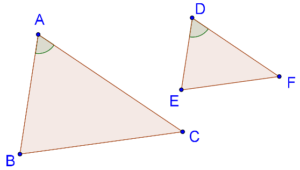It is given that

\begin{align}& \frac{{AB}}{{DE}} = \frac{{AC}}{{DF}} \end{align}

$\angle A = \angle D$

The SAS criterion tells us that $$\Delta ABC$$ ~ $$\Delta DEF$$. Let us see the justification of this.

Proof: Assume that AB > DE. Take a point X on AB such that AX = DE. Through X, draw segment XY parallel to BC, intersecting AC at Y: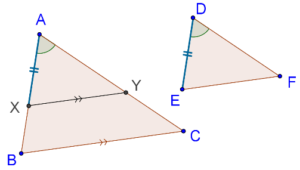Note that $$\Delta AXY$$ ~ $$\Delta ABC$$, and thus:

$\frac{{AX}}{{AB}} = \frac{{AY}}{{AC}}$

Now, we will show that $$\Delta AXY$$ and $$\Delta DEF$$ are congruent. It is given that:

$\frac{{DE}}{{AB}} = \frac{{DF}}{{AC}}$

Since AX = DE, we have:

$\frac{{DE}}{{AB}} = \frac{{AX}}{{AB}} = \frac{{AY}}{{AC}} = \frac{{DF}}{{AC}}$

Thus, AY = DF. By the SAS criterion, $$\Delta AXY$$ ≡ $$\Delta DEF$$. And since $$\Delta AXY$$ ~ $$\Delta ABC$$, this means that $$\Delta DEF$$ and $$\Delta ABC$$ are similar.

Example 1: Consider the following figure: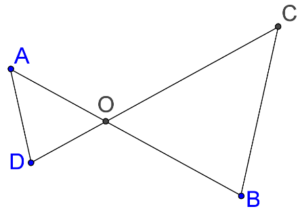Suppose that OA × OB = OC × OD. Show that $$\angle A$$ = $$\angle C$$ and $$\angle B$$ = $$\angle D$$.

Solution: Using the information given to us, we have:

$\frac{{OA}}{{OC}} = \frac{{OD}}{{OB}}$

Thus, two pairs of sides of $$\Delta AOD$$ and $$\Delta COB$$ are respectively proportional. Also, $$\angle AOD$$ = $$\angle COB$$, because these are vertically opposite angles. This means that $$\Delta AOD$$ ~ $$\Delta COB$$, and so:

$$\angle A$$ = $$\angle C$$ and $$\angle B$$ = $$\angle D$$

We can also prove the converse of this. Suppose that it is given to us that $$\angle A$$ = $$\angle C$$ and $$\angle B$$ = $$\angle D$$. Then, $$\Delta AOD$$ and $$\Delta COB$$ are equi-angular, and hence similar. This means that

\begin{align}& \frac{{OA}}{{OC}} = \frac{{OD}}{{OB}}\\& \Rightarrow OA \times OB = OC \times OD \end{align}

Example 2: Consider the following figure: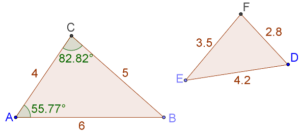Find the value of $$\angle E$$.

Solution: We note that the three sides of the two triangles are respectively proportional:

\begin{align}& \left\{ \begin{gathered}\frac{{DE}}{{AB}} = \frac{{4.2}}{6} = 0.7\\ \frac{{DF}}{{AC}} = \frac{{2.8}}{4} = 0.7\\ \frac{{EF}}{{BC}} = \frac{{3.5}}{5} = 0.7 \end{gathered} \right.\\&\quad\frac{{DE}}{{AB}} = \frac{{DF}}{{AC}} = \frac{{EF}}{{BC}} \end{align}

Thus, the two triangles are similar, which means that they are equi-angular. Note carefully that the equal angles will be:

$\begin{array}{l} \angle A = \angle D = 55.77^0 \\ \angle C = \angle F = 82.82^0 \\ \angle B = \angle E \end{array}$

Finally,

$\begin{array}{l} \angle E = \angle B = 180^0 - (55.77^0 + 82.82^0 )\\ \qquad\qquad\quad= 41.41^0 \end{array}$

Example 3: Consider two similar triangles, $$\Delta ABC$$ and $$\Delta DEF$$: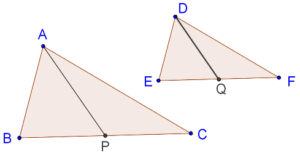AP and DQ are medians in the two triangles respectively. Show that

$\frac{{AP}}{{BC}} = \frac{{DQ}}{{EF}}$

Solution: Since the two triangles are similar, they are equi-angular. This means that $$\angle B$$ = $$\angle E$$. Also,

\begin{align}& \frac{{AB}}{{DE}} = \frac{{BC}}{{EF}}\\& \Rightarrow \quad\frac{{AB}}{{DE}} = \frac{{BC/2}}{{EF/2}} = \frac{{BP}}{{EQ}} \end{align}

Hence, by the SAS criterion, $$\Delta ABP$$ ~ $$\Delta DEQ$$. Thus, the sides of these two triangles will be respectively proportional, and so:

\begin{align}& \frac{{AB}}{{DE}} = \frac{{AP}}{{DQ}}\\& \Rightarrow \quad\frac{{AP}}{{DQ}} = \frac{{BC}}{{EF}}\\& \Rightarrow \quad\frac{{AP}}{{BC}} = \frac{{DQ}}{{EF}} \end{align}

Learn math from the experts and clarify doubts instantly

• Instant doubt clearing (live one on one)
• Learn from India’s best math teachers
• Completely personalized curriculum